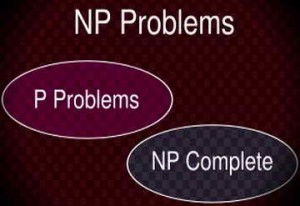# Epistemic Agents In The Context Of The P=?NP ProblemP=?NP is a famous problem common for mathematical logic and mathematical theory of computational complexity. Computational complexity theory divides formal problems in different classes according to the function that describes the output of the computational machine for the given formula as an input. There are several classes that are combined into a complex hierarchy which though has blank spaces.

One of these blank spaces is the relation between classes P and NP. P is the class of problems that can be computed in polynomial time and NP is the class of the problems that can be computed by non-deterministic Turing machine in polynomial time. It is known that NP includes P as a subset but it is not known whether all NP problems are also P or in other words whether P=NP. The problem itself is not at the center of the thesis, instead a minor additional detail is.

The classical method for solving similar problems with complexity classes relations is to relativize the viewed classes to other ones with known properties and prove the required results for them. As shown in literature [Baker T., Gill J., Solovay R. Relativizations of the P=?NP question // SIAM J. Comput. – 1975 – № 4 – Р. 431-442] this method does now work for the P=?NP case as with different contexts it can be proved both positive and negative answer for the posed question. Which on its part brings interesting analogies with the epistemic modal logic.

Epistemic logic is the type of modal logic which formalizes epistemic contexts i.e. what is known and believed by agent. Depending on the particular formal system it can also specify epistemic agent who knows this or that fact. 83 To define non-deterministic Turing machines so-called oracles are used – additional machine tapes that give the answer (“yes” or “no”) whether the language of the initial tape belongs to the computational class or not. This is of course a strong analogy to the epistemic logic agent as it is.

But the analogy gets stronger if we return to the posed problem. It seems that oracles despite giving definite yes-or-no answer do not help with the problem as the relations between classes are complicated. This reminds of the basic modal semantics which operated with worlds combined into different relations according to the accessibility relation.

This further implies that a epistemic modal semantics may be appropriately modified so the worlds will represent computational classes and specified epistemic agents will be Turing machines’ oracles. So the semantics will refer not to states of affairs or truth and lie (in Frege’s sense) but to computations and their complexity affiliations.

As the oracle sometimes cannot give the definite resolution to the computational problem we can theorize about the grades of the competence of epistemic agents in such semantics. Is there is a logically omniscient epistemic agent in such computational semantics? If yes what it means for other agents? What is a relatively competent agent according to the mentioned problems? These are philosophical problems of the minor implication of the famous mathematical issue which deserve further elaboration.

Author Iaroslav Petik